# Area of shapes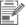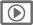a)  draw a shape with an area of 8 square cm.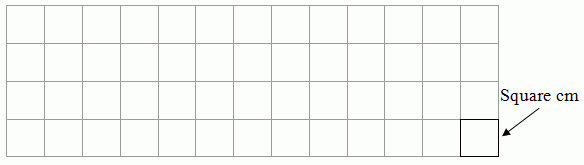b)  Draw a shape with an area of 17 square cm.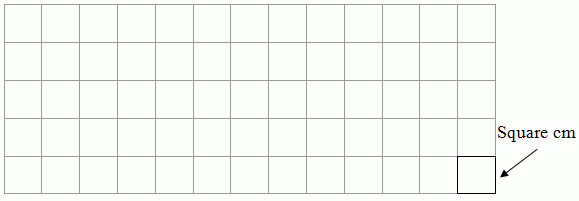## Question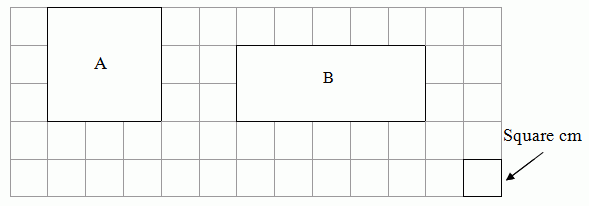c)  i)  Which of these shapes has the bigger area? (Select one)
• A

• B

ii) Explain how you know it is bigger.
This task can be completed with pencil and paper or online.
Level:
2
Curriculum info:
Keywords:
Students draw shapes of given areas, and choose from the bigger area, explaining their reasoning.

Link to National Standards in Measurement

This resource can be used to provide one source of evidence of students' understanding of area.

• Students who use multiplication to work out the areas in part c) shows achievement that is at curriculum level 3 (Year 6).
• Students who draw rectangular or near rectangular arrays in parts a) and b) suggests achievement that is early curriculum level 3 (Year 5).
• Students who use repeated addition or skip counting strategies to work out the areas in part c) and who can draw correct shapes in parts a) and b) suggests achievement at curriculum level 2 (Year 4).
• Students who use counting to work out the areas in part c) and who can draw correct shapes in parts a) and b) suggests achievement that is early curriculum level 2 (Year 3).
• Students who draw shapes that are very close to the correct area in parts a) and b) suggests achievement that is early curriculum level 2 (Year 3).
• Students who can state the bigger shape in part c) with an incorrect or with no justification suggests achievement at curriculum level 1 (Year 2).

Students who drew shapes that were rectangular, or close to rectangular, had significantly higher mean abilities than students who drew other shapes.

Learning Progression Frameworks
This resource can provide evidence of learning associated with within the Mathematics Learning Progressions Frameworks.
 Y4 (10/2010) a) Draws 8 unit squares. - Give credit for students who use other drawings with an area of 8 square cm. - Do NOT accept responses where several disconnected shapes are drawn. - Do NOT give credit if squares of different sizes are drawn. easy easy b) Draws 17 unit squares. - Give credit for students who use other drawings with an area of 17 square cm. - Do NOT accept responses where several disconnected shapes are drawn. - Do NOT give credit if squares of different sizes are drawn. moderate moderate c) B  Any 1 of the following: B is 5 × 2 = 10 but A is 3 × 3 = 9; B has 10 squares but A has 9; B has more squares than A; States they counted all the squares; Reasonable attempt to state the relative proportions of the shapes; If you cut it (B) in half then put one half on top it would be taller. States that B has 10 squares (or A has 9). Look for visual clues, such as marks around the perimeter, or dots in the boxes as they were counted. B and correct explanation easy difficult                     difficult
Teaching and learning:

This resource looks at students' ability to use unit squares as measure of area. They do this firstly by constructing areas of given sizes, and then chose and justify which of two shapes has the biggest area. There is the potential for multiplicative strategies, either multiplying rows by columns or repeated addition/skip counting.

Diagnostic and formative information:
 Likely reason a) b) 7 or 9 16 or 18 Miscounts the squares These students drew one square too few or too many. c) Uses only width (length) or height B - Because longer is bigger than fatter. A - Fatter is bigger. B – Because if you turn it, it will be taller. And if you leave it, it will be wider. B – Because it is wider than A. c) Sees area as a property of the shape A – [A] square is bigger than a rectangle. B – Because … rectangles are bigger than squares. c) Confuses area and perimeter B – Because you count how many squares are next to it. B - It has a wide space around it. B – Because I counted the little boxes around it and B was the biggest number. Look for visual clues, such as marks around the perimeter.
Next steps:

Miscounts the squares
Get the student to confirm their answer. If they can see that it is incorrect, then they are probably achieving at curriculum level 1, but need to learn to work more carefully and to check their work after it is completed.
If their answer is one square too few in a) and b), ask them to justify it. If they say "There already is one square there" they are probably achieving at curriculum level 2.

Uses only width (length) or height or sees area as a property of the shape
These students may well be estimating the area visually, which is a Level 1 approach. They need to move on to measuring the area by counting unit squares. Their ideas could be challenged by drawing a 3 × 3 square and a 1 × 8 or 1 × 10 rectangle and asked "Which is bigger?" A discussion could lead to the conclusion that they would have to count the unit squares to be sure.

Confuses area and perimeter
The meaning of the words "perimeter" and "area" is a knowledge issue. Students need to see both concepts are valid measures, but they measure different things.

• Perimeter measures the length of the distance around. This can be counted using unit lengths (counting from one on materials), or by adding the lengths of each side.
• Area measures the "space" covered on a flat surface. Analogues such as "How much paint is needed?" or "Which one will take longer to colour in?" may be useful.

Part c) asks for the bigger shape. This implies the biggest size of the shape, and size of a flat shape is measured in area. You could say to the student "You have given the shape which has the bigger distance around it. This is called the perimeter. Can you tell me which shape has the bigger area?"